# 1 Installation

You can install codacore by running:

install.packages("codacore")

You may instead install the development version directly from Github, using the devtools package.

devtools::install_github("egr95/R-codacore", ref="main")

Note that CoDaCoRe requires a working installation of TensorFlow. If you do not have Tensorflow previously installed, when you run codacore() for the first time you will likely encounter an error message of the form:

> codacore(x, y)

ERROR: Could not find a version that satisfies the requirement tensorflow
ERROR: No matching distribution found for tensorflow

Python environments searched for 'tensorflow' package:
/moto/stats/users/eg2912/miniconda3/envs/r-test/bin/python3.9
/usr/bin/python2.7

You can install TensorFlow using the install_tensorflow() function.

This can be fixed simply by installing tensorflow, as follows:

install.packages("tensorflow")
library("tensorflow")
install_tensorflow()

install.packages("keras")
library("keras")
install_keras()

Note also that you may have to restart your R session between installation of codacore, tensorflow, and keras.

# 2 Summary of method

CoDaCoRe is an algorithm to identify predictive log-ratio biomarkers in high-throughput sequencing data. Let $$x$$ denote HTS input (e.g., $$x_{i,j}$$ denotes the abundance of the $$j$$th bacteria in the $$i$$th subject), and let $$y$$ denote the outcome of interest (e.g., $$y_i$$ is equal to 0 or 1 depending on whether the $$i$$th subject belonged to the case or the control group). Given a set of $$(x_i, y_i)$$ pairs, CoDaCoRe identifies predictive biomarkers of the form: $B(x_i; J^+, J^-) = \log \left( \frac{\sum_{j \in J^+} x_{i,j}}{\sum_{j \in J^-} x_{i,j}} \right),$ that are maximally associated with the response variable $$y_i$$. In other words, CoDaCoRe identifies a numerator set $$J^+$$ and a denominator set $$J^-$$, such that their log-ratio is most predictive of the response variable. By default, CoDaCoRe uses balances, which are defined as the log-ratio of geometric means (as opposed to summations): $B(x_i; J^+, J^-) = \log \left( \frac{(\prod_{j \in J^+} x_{i,j})^{|J^+|}}{(\prod_{j \in J^-} x_{i,j})^{|J^-|}} \right).$

For an introduction to balances, we refer the reader to the selbal paper, and for a more detailed treatment of CoDaCoRe and other log-ratio methodology, we refer the reader to the codacore paper and this paper.

# 3 Training the model

We assume a working installation of codacore (link).

library("codacore")
help(codacore)

In this tutorial, we will showcase codacore using three datasets that were also analyzed by the authors of selbal (Rivera-Pinto et al., 2018). First, we consider the Crohn’s disease data from (Gevers et al., 2014).

data("Crohn")
x <- Crohn[, -ncol(Crohn)]
y <- Crohn[, ncol(Crohn)]

Our goal is to identify ratio-based biomarkers that are predictive of disease status. Our input variable consists of the abundance of 48 microbial species in 975 samples. As is common in most machine learning libraries, our package expects an input of shape (n, p), with a row for each sample and a column for each variable.

dim(x)
#>  975  48

The output variable is a binary indicator (CD stands for Chron’s disease).

table(y)
#> y
#>  CD  no
#> 662 313

Prior to fitting CoDaCoRe, we must impute any zeros in our input variable (a standard pre-processing step for ratio-based methods).

x <- x + 1

Next, we split our data into a training and a test set (to keep things simple we do this naively at random, though in practice one might consider stratified sampling and class rebalancing).

# For reproducibility, we set a random
# seed (including in TensorFlow, used
# by codacore)
set.seed(0)
library(tensorflow)
tf$random$set_seed(0)
trainIndex <- sample(1:nrow(x), 0.8 * nrow(x))
xTrain <- x[trainIndex, ]
yTrain <- y[trainIndex]

We are ready to fit CoDaCoRe. We stick to the default parameters for now. Notice the fast runtime (as compared to, for example, selbal.cv).

model <- codacore(
xTrain,
yTrain,
logRatioType = 'balances', # can also use 'amalgamations'
lambda = 1                 # regularization parameter (1 corresponds to "1SE rule")
)

# 4 Visualizing results

Next we can check the learned output of the model: what inputs were included in the learned log-ratios, how strongly associated they are to the response, and how well they classified the data.

print(model)
#>
#> Number of log-ratios found: 2
#> ***
#> Log-ratio rank 1
#> Numerator: g__Roseburia o__Clostridiales_g__
#> Denominator: g__Dialister g__Aggregatibacter
#> AUC: 0.7567907
#> Slope: 0.3939618
#> ***
#> Log-ratio rank 2
#> Numerator: g__Parabacteroides f__Peptostreptococcaceae_g__ g__Bacteroides g__Faecalibacterium g__Roseburia g__Lachnospira o__Clostridiales_g__ f__Rikenellaceae_g__
#> Denominator: g__Eggerthella g__Dialister g__Streptococcus g__Aggregatibacter
#> AUC: 0.778969
#> Slope: 0.3002488

The most predictive ratio identified by CoDaCoRe is Roseburia / Dialister, which can be visualized with the plot function.

plot(model)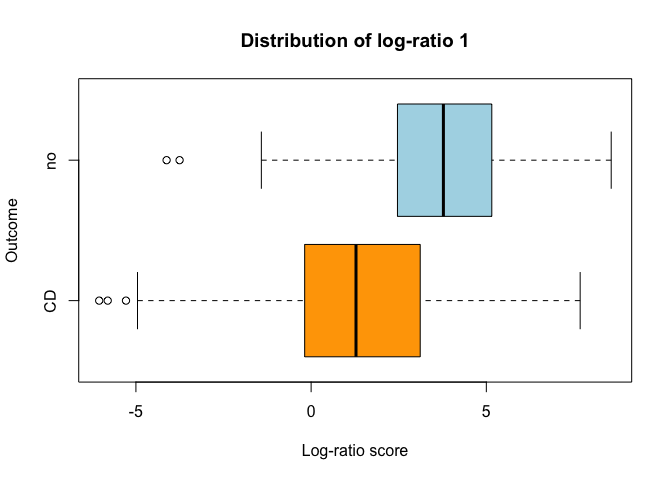Note that CoDaCoRe is an ensemble model, where multiple log-ratios are learned sequentially in decreasing order of importance (with automatic stopping whenever no additional log-ratio improved the loss function during training). We can visualize the performance of this ensembling procedure by “stacking” the respective ROC curves.

plotROC(model)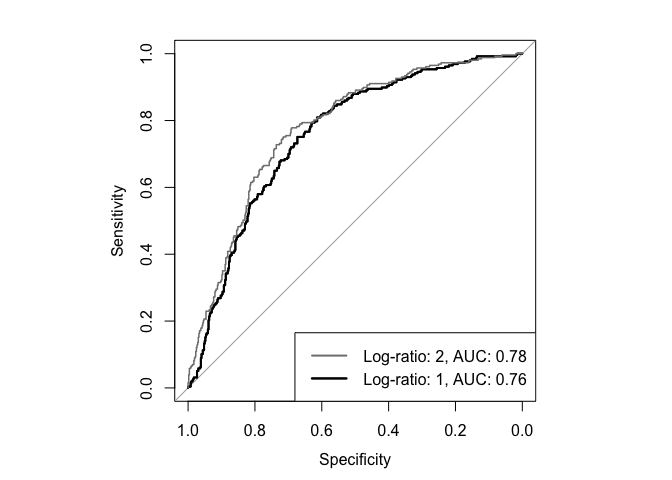# 5 Predicting on new data

We can also use our trained model to classify new samples.

xTest <- x[-trainIndex, ]
yTest <- y[-trainIndex]
yHat <- predict(model, xTest, logits = F)
cat("Test set AUC =", pROC::auc(pROC::roc(yTest,
yHat, quiet = T)))
#> Test set AUC = 0.80537
# Convert probabilities into a binary
# class
failure <- yHat < 0.5
success <- yHat >= 0.5
yHat[failure] <- levels(y)
yHat[success] <- levels(y)
cat("Classification accuracy on test set =",
round(mean(yHat == yTest), 2))
#> Classification accuracy on test set = 0.73

Note our predict function can be restricted to only use the top k log-ratios in the model for prediction. For example, the following will compute the AUC of a 1-log-ratio model, using only the top log-ratio.

yHat <- predict(model, xTest, logits = F,
numLogRatios = 1)
cat("Test set AUC =", pROC::auc(pROC::roc(yTest,
yHat, quiet = T)))
#> Test set AUC = 0.7859712

Other useful functions include:

getNumeratorParts(model, 1)
getDenominatorParts(model, 1)
getLogRatios(model, xTest)
getNumLogRatios(model)
getTidyTable(model)
getSlopes(model)

# 6 Controlling overlap between log-ratios

By default, CoDaCoRe allows for “overlapping log-ratios”, in other words, an input variable that is included in the first log-ratio may well be included in a second or third log-ratio provided it is sufficiently predictive. However, the user may choose to restrict each successive log-ratio to be constructed from a mutually exclusive set of input variables (e.g., to obtain orthogonal balances, in the Aitchison sense). This can be specified with the parameter overlap. In our example, note how g__Dialister is no longer repeated.

model <- codacore(xTrain, yTrain, overlap = F)
print(model)
#>
#> Number of log-ratios found: 2
#> ***
#> Log-ratio rank 1
#> Numerator: g__Roseburia o__Clostridiales_g__
#> Denominator: g__Dialister g__Aggregatibacter
#> AUC: 0.7567907
#> Slope: 0.3939618
#> ***
#> Log-ratio rank 2
#> Numerator: g__Parabacteroides f__Peptostreptococcaceae_g__ g__Bacteroides g__Faecalibacterium g__Lachnospira f__Rikenellaceae_g__
#> Denominator: g__Eggerthella f__Enterobacteriaceae_g__
#> AUC: 0.7712166
#> Slope: 0.1858207

# 7 Using amalgamations (summed-log-ratios)

CoDaCoRe can be used to learn log-ratios between both geometric means (known as “balances” or “isometric-log-ratio”) or summations (known as “amalgamations” or “summed-log-ratio”), depending on the goals of the user. This can be specified with the parameter logRatioType.

model <- codacore(xTrain, yTrain, logRatioType = "amalgamations")
print(model)
#>
#> Number of log-ratios found: 1
#> ***
#> Log-ratio rank 1
#> Numerator: g__Parabacteroides g__Bacteroides g__Faecalibacterium o__Clostridiales_g__
#> Denominator: g__Haemophilus g__Blautia f__Enterobacteriaceae_g__ g__Dialister g__Streptococcus g__Fusobacterium
#> AUC: 0.7104552
#> Slope: 0.4062366

Note that amalgamations/summed-log-ratios are less sensitive to covariates that are small in magnitude (e.g., rare microbes), which can hinder their predictive strength for datasets where small covariates are important. On the other hand, summed-log-ratios have a different interpretation than isometric-log-ratios and may therefore be preferrable in some applications (e.g., when the “summed” effect of an aggregated sub-population is the object of interest). In our Crohn’s disease data, the rare species Roseburia gets picked up by the isometric-log-ratio, but not by the summed-log-ratio, which is more sensitive to more common bacteria species such as Faecalibacterium.

# 8 Continuous outcomes

We consider the HIV data from (Noguera-Julian et al., 2016). The goal here is to construct a log-ratio of the microbial abundances that is predictive of the inflammation marker “sCD14”, a continuous response variable. CoDaCoRe can be applied much in the same way, except the loss function changes from binary cross-entropy to mean-squared-error. This change will happen automatically based on the values inputted as y (although it can also be overriden manually via the objective parameter, for example, if the user wanted to fit a binary response using the mean-squared-error, they could specify objective = 'regression').

data("sCD14")
x <- sCD14[, -ncol(sCD14)]
y <- sCD14[, ncol(sCD14)]

# Replace zeros as before
x <- x + 1

# Split the data
trainIndex <- sample(1:nrow(x), 0.8 * nrow(x))
xTrain <- x[trainIndex, ]
yTrain <- y[trainIndex]

# Fit codacore and inspect results
model <- codacore(xTrain, yTrain)
print(model)
#>
#> Number of log-ratios found: 1
#> ***
#> Log-ratio rank 1
#> Numerator: g_Subdoligranulum
#> Denominator: g_Bifidobacterium
#> R squared: 0.1438051
#> Slope: 611.0956
plot(model)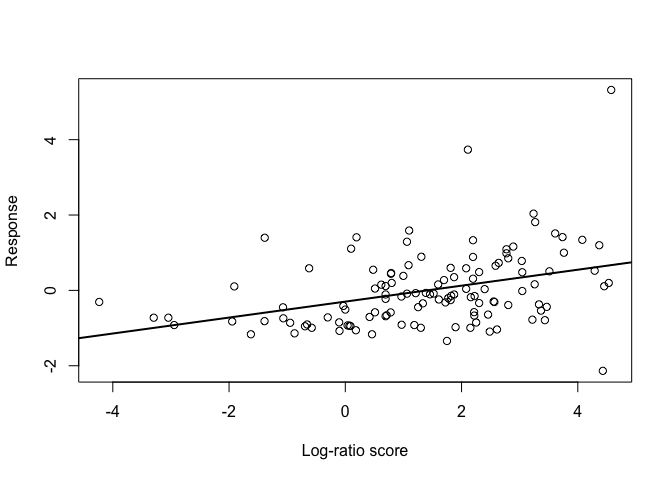# 9 Multiclass classification

Our implementation does not currently support multiclass targets, however a multiclass classifier can be constructed from CoDaCoRe by taking the One-vs-One or One-vs-Rest strategies.

# 10 Tuning the regularization parameter lambda

The parameter lambda controls the regularization strength of CoDaCoRe. In particular, lambda = 1 (the default value) corresponds to applying the 1-standard-error rule in the discretization step of the log-ratio (details in Section 3.3). This is typically a good choice, leading to models that are both sparse and predictive. Sparser models can be achieved by higher values of lambda, for example, lambda = 2 corresponds to applying a “2-standard-error” rule. On the other hand, smaller values of lambda result in less sparse, but typically most predictive, models. In particular, lambda = 0 corresponds to a “0 standard-error rule”, in other words choosing the log-ratio that minimizes cross-validation score. Such a choice can be good when we seek a maximally predictive model, but care less about sparsity.

model <- codacore(xTrain, yTrain, lambda = 0)
print(model)
#>
#> Number of log-ratios found: 3
#> ***
#> Log-ratio rank 1
#> Numerator: g_Subdoligranulum g_Dialister g_Paraprevotella f_Defluviitaleaceae_g_Incertae_Sedis
#> Denominator: f_Lachnospiraceae_g_unclassified g_Succinivibrio g_Parabacteroides g_Lachnospira g_Coprococcus g_Catenibacterium g_Roseburia g_Megasphaera g_Mitsuokella g_Sutterella g_Bifidobacterium g_Streptococcus f_Erysipelotrichaceae_g_unclassified f_Porphyromonadaceae_g_unclassified g_Collinsella
#> R squared: 0.319985
#> Slope: 2040.222
#> ***
#> Log-ratio rank 2
#> Numerator: g_Faecalibacterium g_Bacteroides g_Alloprevotella g_Subdoligranulum g_Clostridium_sensu_stricto_1 g_Escherichia-Shigella f_Defluviitaleaceae_g_Incertae_Sedis
#> Denominator: f_Lachnospiraceae_g_unclassified g_Lachnospira g_Barnesiella g_Catenibacterium g_Megasphaera g_Mitsuokella g_Sutterella g_Bifidobacterium g_Collinsella
#> R squared: 0.3852063
#> Slope: 816.7075
#> ***
#> Log-ratio rank 3
#> Numerator: g_Alloprevotella
#> Denominator: g_Bifidobacterium
#> R squared: 0.390754
#> Slope: 66.46405

Notice the increased R-squared score relative to the previous model (at the expense of sparsity).

## 10.1 When no predictive log-ratios are found

On some datasets, CoDaCoRe may have trouble finding any predictive log-ratios. If none are found, this is typically a sign that the signal in the data is weak. In this case, the analyst may choose to reduce the value of lambda (for example, to lambda = 0), in order to allow our algorithm to search more aggressively for predictive log-ratios. Doing so will often allow the algorithm to identify at least one predictive log-ratio, at the risk of overfitting the training data. Additional care must be taken in validating such log-ratios on held-out data.

# 11 Covariate adjustment

Many applications require accounting for potential confounder variables as well as our ratio-based biomarkers. As an example, we consider a second HIV dataset from (Noguera-Julian et al. 2016). The goal is to find a microbial signature for HIV status, i.e., a log-ratio that can discriminate between HIV-positive and HIV-negative individuals. However, we have an additional confounder variable, MSM (Men who have Sex with Men). In the context of CoDaCoRe, there are multiple approaches that can be used to adjust for covariates.

## 11.1 Incremental fit

Given the stagewise-additive (i.e., ensemble) nature of CoDaCoRe, whereby each successive log-ratio is fitted on the residual of the previous iteration, a very natural approach is to fit the covariates a priori and then fit CoDaCoRe on the residual. In other words, we would start by regressing HIV status on MSM, “partialling out” this covariate, and then fit CoDaCoRe on the residual from this model. This can be implemented easily by means of the offset parameter.

data("HIV")
x <- HIV[, 1:(ncol(HIV) - 2)]
z <- HIV[, "MSM"]
y <- HIV$HIV_Status # Replace zeros as before x <- x + 1 # Split the data trainIndex <- sample(1:nrow(x), 0.8 * nrow(x)) dfTrain <- HIV[trainIndex, ] xTrain <- x[trainIndex, ] yTrain <- y[trainIndex] partial <- glm(HIV_Status ~ MSM, data = dfTrain, family = "binomial") # Note the offset must be given in # logit space model <- codacore(xTrain, yTrain, offset = predict(partial)) print(model) #> #> Number of log-ratios found: 1 #> *** #> Log-ratio rank 1 #> Numerator: g_Bacteroides g_Anaerovibrio #> Denominator: g_Prevotella g_Alloprevotella g_RC9_gut_group g_Catenibacterium g_Dialister f_Ruminococcaceae_g_Incertae_Sedis f_vadinBB60_g_unclassified g_Oribacterium #> AUC: 0.8019231 #> Slope: 0.5233792 partialAUC <- pROC::auc(pROC::roc(yTrain, predict(partial), quiet = T)) codacoreAUC <- model$ensemble[]$AUC cat("AUC gain:", round(100 * (codacoreAUC - partialAUC)), "%") #> AUC gain: 16 % Note that, when predicting on new data, the contributions of the covariates and the log-ratios should be added up in logit space. dfTest <- HIV[-trainIndex, ] xTest <- x[-trainIndex, ] yTest <- z[-trainIndex] yHatLogit <- predict(partial, newdata = dfTest) + predict(model, xTest, logits = T) yHat <- yHatLogit > 0 # in case we need binary predictions e.g. to compute accuracy testAUC <- pROC::auc(pROC::roc(yTest, yHatLogit, quiet = T)) cat("Test AUC:", round(100 * testAUC), "%") #> Test AUC: 100 % When the outcome variable is continuous, this is simpler as there is no logit transformation and the contributions of the partial model can be added directly, e.g., # Suppose that, instead of predicting HIV status (a binary target), # we now have some continuous target, 'yCts' partial2 <- lm(yCts ~ MSM, data=dfTrain) model2 <- codacore(xTrain, yCtsTrain, offset=predict(partial)) print(model2) yCtsHat <- predict(partial2, newdata = dfTest) + predict(model2, xTest) MSE <- mean((yCtsTest - yCtsHat)^2) ## 11.2 Joint fit Depending on the application and the goals of the analyst, it may be of interest to understand the joint effect of the covariates and log-ratios on the response. To do so, one option is to simply regress the outcome jointly against the covariates and the learned log-ratios from the previous step. This can be implemented by running, in addition to the above, an additional glm fit. # Create a new design matrix with # response & covariates, as well as # log-ratios obtained from codacore dfJoint = cbind(dfTrain[, c("MSM", "HIV_Status")], getLogRatios(model)) # And fit everything jointly modelJoint <- glm(HIV_Status ~ ., data = dfJoint, family = "binomial") # Can again use this model to make # predictions or to interpret # regression coefficients yHat <- predict(modelJoint, newData = dfJoint) summary(modelJoint) #> #> Call: #> glm(formula = HIV_Status ~ ., family = "binomial", data = dfJoint) #> #> Deviance Residuals: #> Min 1Q Median 3Q Max #> -2.6502 0.1875 0.3587 0.6123 1.1661 #> #> Coefficients: #> Estimate Std. Error z value Pr(>|z|) #> (Intercept) 1.1801 0.7053 1.673 0.094268 . #> MSMMSM 0.6863 0.8905 0.771 0.440916 #> log-ratio1 0.7671 0.2226 3.446 0.000568 *** #> --- #> Signif. codes: 0 '***' 0.001 '**' 0.01 '*' 0.05 '.' 0.1 ' ' 1 #> #> (Dispersion parameter for binomial family taken to be 1) #> #> Null deviance: 109.567 on 123 degrees of freedom #> Residual deviance: 88.559 on 121 degrees of freedom #> AIC: 94.559 #> #> Number of Fisher Scoring iterations: 6 Note that, in any case, the CoDaCoRe algorithm itself only optimizes over one log-ratio at a time (in its current implementation). In some applications, it may in fact be beneficial to optimize over the set of log-ratios jointly with the regression coefficients of the covariates. However, this is not yet implemented. # 12 Unsupervised learning CoDaCoRe can be used as follows to obtain a fast, scalable, interpretable and sparse log-ratio based unsupervised learning algorithm. The idea is to first compute a dense representation of the data using traditional methods, and then regress the data against this representation using CoDaCoRe to obtain a sparse log-ratio representation in its stead. For example, one could take the first principal component of the CLR-transformed data, and use CoDaCoRe to approximate this real-valued representation with a single sparse log-ratio score (Quinn et al., 2021). In the present HIV dataset, we find that the learned log-ratio biomarker provides a useful representation of the data, markedly separating the MSM from the non-MSM individuals. clr <- t(apply(x, 1, function(x) log(x) - mean(log(x)))) pca <- prcomp(clr, scale = T) pc1 = clr %*% pca$rotation[, 1]

model <- codacore(x, as.numeric(pc1))
logRatio1 <- getLogRatios(model, x)[, 1]
boxplot(logRatio1 ~ z)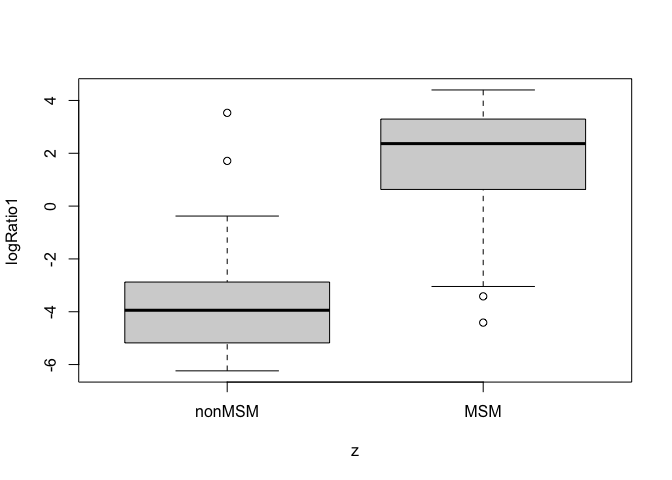We can take things one step further and derive a second unsupervised log-ratio biomarker, by simply fitting CoDaCoRe on the second principal component. Taken together, our two log-ratio biomarkers capture important information in the data:

pc2 = clr %*% pca$rotation[, 2] model <- codacore(x, as.numeric(pc2)) logRatio2 <- getLogRatios(model, x)[, 1] plot(logRatio1, logRatio2, col = z) legend("bottomleft", legend = levels(z), pch = 1, col = 1:2)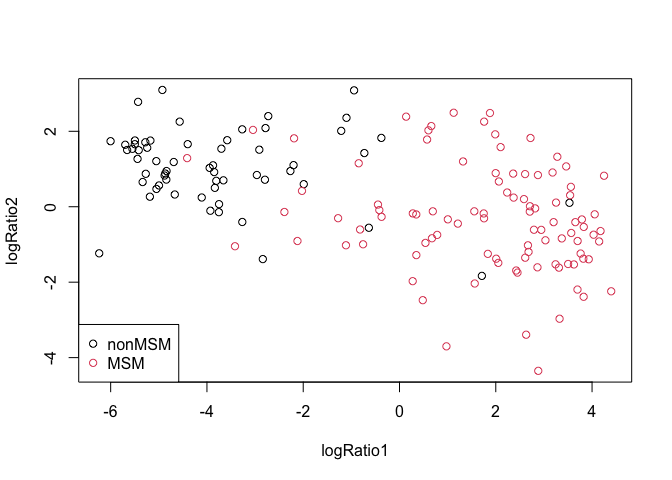Note also that the CoDaCoRe framework can be applied to the unsupervised learning problem in several other ways, some of which are under active development. # 13 Multi-omics integration With a similar approach, CoDaCoRe can be used for scalable, sparse, and interpretable multi-omics data integration. We briefly highlight an example multi-omics analysis of paired gut microbiome and metabolomics data, taken from 220 clinical samples of which 88 have Chron’s disease and 76 have ulcerative colitis (Franzosa et al., 2019). For a full analysis, see Section 5 and the appendix in Quinn et al., 2021. Again, we will use standard techniques to compute a (dense) latent representation of the data, which we will then approximate using sparse log-ratio biomarkers. Letting $$\mathbf T$$ denote the microbe abundances $$\mathbf U$$ the metabolite abundances, we will use partial least squares (PLS) regression to model the association between $$\mathbf T$$ and $$\mathbf U$$. This will result in two latent factors, one for $$\mathbf T$$ and one for $$\mathbf U$$, that capture the joint information in the data. These latent factors will constitute the regression target for CoDaCoRe. # Load data download.file("https://github.com/egr95/FranzosaData/blob/main/FranzosaMicrobiome.rda?raw=true", "FranzosaMicrobiome") download.file("https://github.com/egr95/FranzosaData/blob/main/FranzosaMetabolite.rda?raw=true", "FranzosaMetabolite") load("FranzosaMicrobiome") load("FranzosaMetabolite") # Note data have already been # pre-processed as per (Quinn et al., # 2021), including zero-replacement and # normalization to a unit total. T <- FranzosaMicrobiome[, -ncol(FranzosaMicrobiome)] # We remove the last column (response variable) U <- FranzosaMetabolite[, -ncol(FranzosaMetabolite)] # Apply clr transform prior to PLS clrT <- t(apply(T, 1, function(x) log(x) - mean(log(x)))) clrU <- t(apply(U, 1, function(x) log(x) - mean(log(x)))) # Call mixOmics package and plot first # PLS components suppressMessages(library("mixOmics")) pls <- mixOmics::pls(X = clrT, Y = clrU, ncomp = 1) plot(pls$variates$X[, 1], pls$variates$Y[, 1], main = "PLS multi-omics (dense)")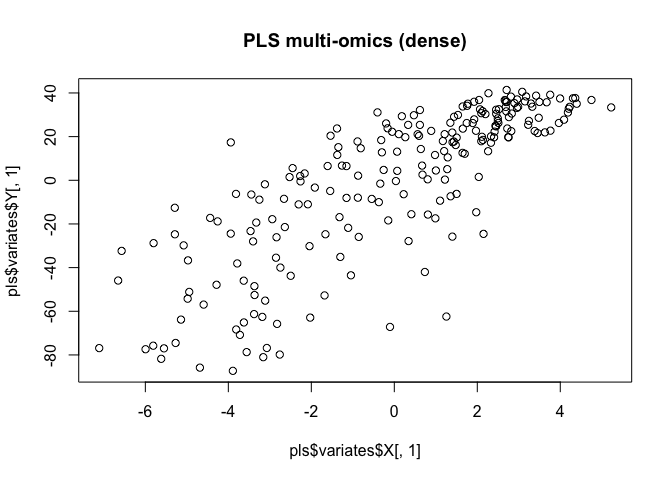# Approximate the dense PLS # representations with sparse log-ratio # biomarkers plsX <- pls$variates$X[, 1] modelX <- codacore(T, plsX) logRatioX <- getLogRatios(modelX)[, 1] plsY <- pls$variates\$Y[, 1]
modelY <- codacore(U, plsY, logRatioType = "B")
logRatioY <- getLogRatios(modelY)[, 1]

plot(logRatioX, logRatioY, main = "CoDaCoRe multi-omics (sparse)")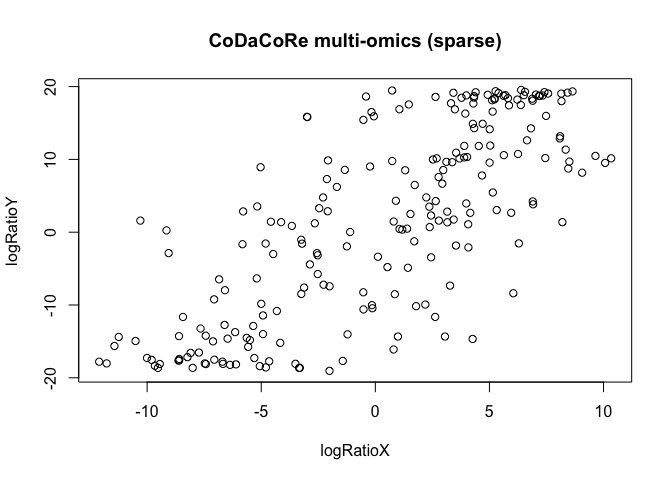Note that CoDaCoRe obtains a sparse representation that also has better statistical properties than the original (dense) PLS components, markedly de-skewing the data.